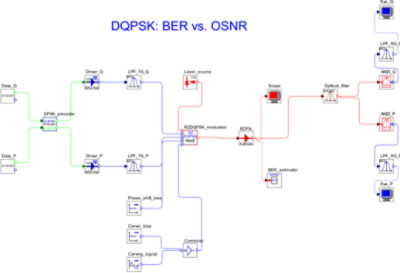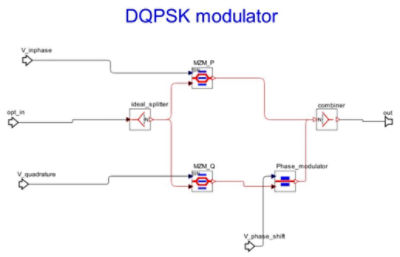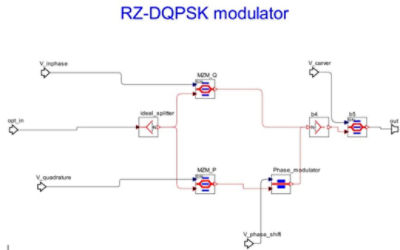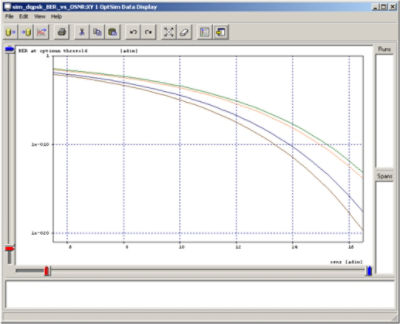# DQPSK Modulation: BER curves as a function of OSNR

## Tool Used: OptSim

These examples refer to the so-called sensitivity analysis for DQPSK, RZ-DQPSK and CSRZ-DQPSK modulation formats, shown in OptSim. It means that such examples give the curve of BER vs. the OSNR measured at the receiver. As OSNR we define the Optical Signal-to-Noise Ratio considering the amount of noise measured on an equivalent noise bandwidth equal to the bit-rate. The analysis is carried out using the semi analytical BER estimator based on the Karhunen-Loewe series expansion of noise.

The layout looks like:For standard DQPSK (Non Return to Zero) the DQPSK modulator is used. Its internal layout is described in figure below. It can be observed that the optical source is split, and the two signal components are modulated through amplitude modulators by the in-phase and quadrature data flows. The quadrature signal is properly phase shifted by the phase modulator driven by the phase -shift bias.The following diagram shows DQPSK modulator for the RZ case:The figure below shows BER vs. OSNR for the four different "flavors" of DQPSK modulation: